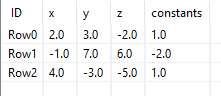# Solving a system of linear equations using KNIME

Hello,
I have a set of equations of the form (or close to this form):

(1) A = Bx + Cy
(2) D = Ez + Fy
(3) G = Ht + Iy

(n) 1 = x + y + z + t + …

Some complicating factors:

• The number of equations is flexible but I believe that it will always be a linear system.
• The equality is actually a range (ie 0 <= A <= 1, 0.5 <= D <= 1.5, etc.) and any solution within the range which satisfies the system is valid.
• The number of terms in each linear equation is variable.
• There is no guarantee a solution exists.
• I’m 99% sure these will all boil down to a linear system BUT if it turns out to be non-linear what is the play?

All capital letters are constants or knowns and the lower case letters are variables. What is the best way to import this into KNIME and solve for the variables?
A colleague suggested looking at the optimization workflow examples in KNIME but I figured I’d also post this question since I didn’t see it on the forum.

Thanks,
Dseller

Hello @dseller,

to my knowledge there are no dedicated nodes for your task. Workaround is to go with R/Python integrations…

Br,
Ivan

Thanks @ipazin,
Do you know of any good example workflows solving something like this using the python node(s)?

Hello @dseller,

no unfortunately. But you can search KNIME Hub. And also maybe someone else joins discussion and provides useful idea/suggestionsBr,
Ivan

Hi.
One approach would be to leverage the Java library Apache commons math 3 in a Java Snippet node.
See Math – The Commons Math User Guide - Linear Algebra
One can add the library under the “Additional Bundles” tab of the Java Snippet configuration dialog.

I threw together a quick example that allows one to specify the columns containing equation terms, as well the column containing the constants. The solution (if there is one) is output as a variable called coefficients (a comma-separated string of doubles (currently, variables of type double array aren’t fully supported in version 4.3)). The corresponding column names are output as a variable called termColsString. The input columns must be of type double. The example attached solves the following system of linear equations:
2x + 3y - 2z = 1
-x + 7y + 6x = -2
4x - 3y - 5z = 1
Here’s the corresponding input table:and the output variables:Sorry I don’t have time to add refinements like error checking or gracefully alerting when there is no solution, etc. Also, I haven’t had time to extensively test the solution. In any case, hope this helps / gets you started.
-Don
solving system of linear eqns.knwf (21.7 KB)

2 Likes

Hi @dnaki,
Thanks for the example. I am sure I can get something like this to work for me.

Do you know of any online Java guides/explanations so I can start to figure out your code? I have zero experience with Java.

Hi @dseller ,
Apologies, but it’s been many years since I learned Java and I’m afraid I’m not familiar with current online learning resources.
-Don

@dnaki Is there an easy way to restrict the answers to 0<=x<=1?

Thanks

This topic was automatically closed 182 days after the last reply. New replies are no longer allowed.